# 如何使用libgdx编写一个简单的游戏（一）— 雏形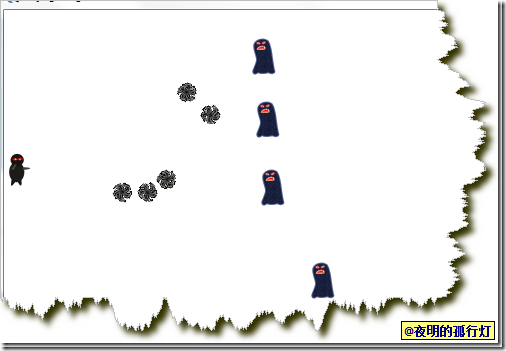# 获取libgdx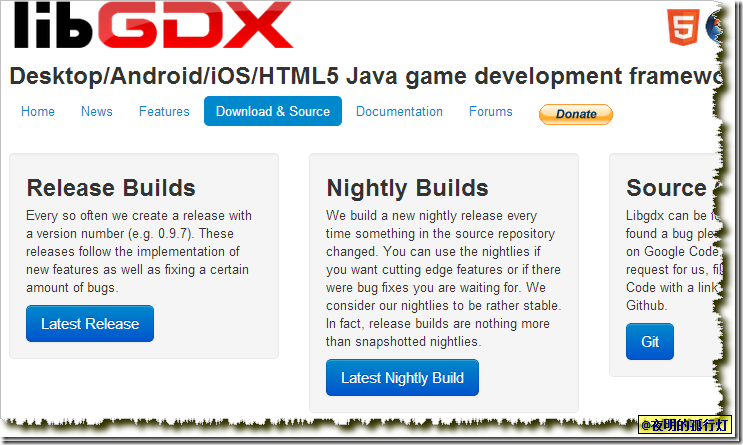# 创建项目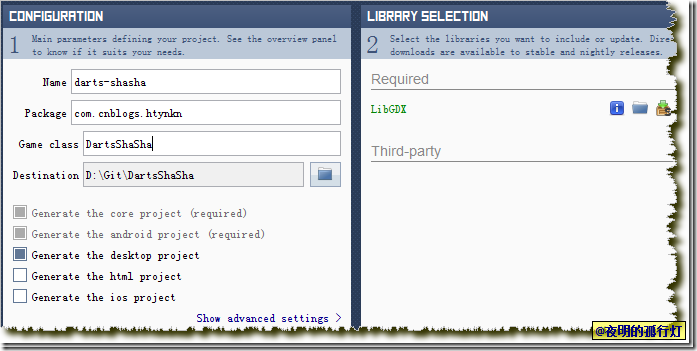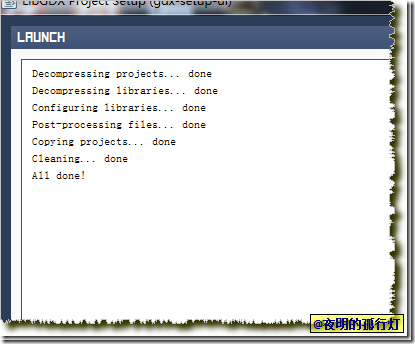# 准备工作```package com.cnblogs.htynkn;

public class DartsShaSha extends ApplicationAdapter {
Stage stage;
@Override
public void create() {
stage = new Stage(480, 320, true);
}

@Override
public void dispose() {
stage.dispose();
}

@Override
public void render() {
Gdx.gl.glClearColor(1, 1, 1, 1);
Gdx.gl.glClear(GL10.GL_COLOR_BUFFER_BIT);
stage.act();
stage.draw();
}
}```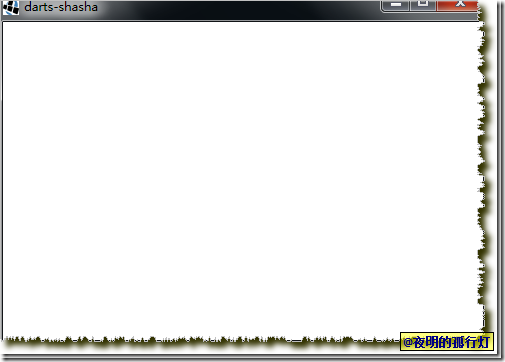`stage = new Stage(480, 320, true);`

```LabelStyle labelStyle = new LabelStyle(new BitmapFont(), Color.BLACK); //创建一个Label样式，使用默认黑色字体
Label label = new Label("FPS:", labelStyle); //创建标签，显示的文字是FPS：
label.setName("fpsLabel"); //设置标签名称为fpsLabel
label.setY(0); //设置Y为0，即显示在最下面
label.setX(480 - label.getTextBounds().width); //设置X值，显示为最后一个字紧靠屏幕最右侧

```Label label = (Label) stage.getRoot().findActor("fpsLabel"); //获取名为fpsLabel的标签
label.setText("FPS:" + Gdx.graphics.getFramesPerSecond());
label.setX(480 - label.getTextBounds().width); //更新X值以保证显示位置正确性```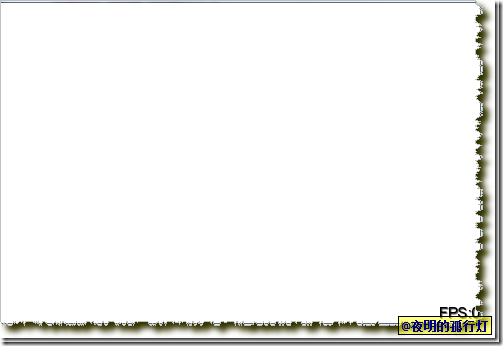# 添加忍者

`TextureAtlas atlas = new TextureAtlas("pack/default.pack");`

```Image man = new Image(atlas.findRegion("Player")); //获取图册中的Player.png并创建image对象
man.setX(0);
man.setY(160 - man.getHeight() / 2); //设置Y值，以让图片在中间显示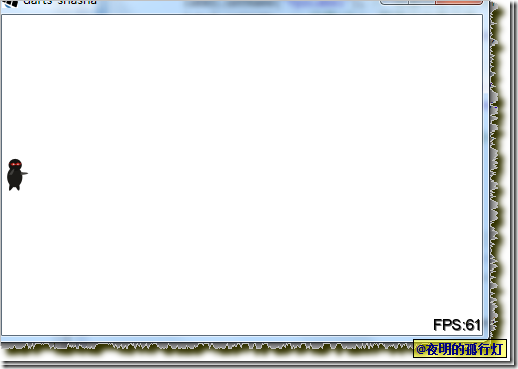# 添加怪兽

```package com.cnblogs.htynkn;

public class TargetGroup extends Group {
public TargetGroup(AtlasRegion region) {
super();
}
}```

```int minY = 0;
int maxY = (int) (320 - region.getRegionHeight());
int tempY = MathUtils.random(minY, maxY);```

```int tempY = 0;
for (int i = 0; i < 3; i++) {
Image image = new Image(region);
image.setX(480 - image.getWidth());
// 开始判断Y值是否符合要求
boolean flag = false;
do {
flag = false;
tempY = MathUtils.random(minY, maxY); // 生成Y值

Actor[] actors = this.getChildren().begin(); // 获取当前已有的怪兽对象
for (int j = 0; j < this.getChildren().size; j++) {
Actor tempActor = actors[j];
if (tempY == tempActor.getY()) { // 如果Y值相等，比如重合，所以舍弃,重新生成
flag = true;
break;
} else if (tempY < tempActor.getY()) { // 如果生成的Y值小于当前怪兽的Y值，则判断生成的Y值加上高度后是否合适
if ((tempY + region.getRegionHeight()) >= tempActor
.getY()) {
flag = true;
break;
}
} else { // 如果生成的Y值大于当前怪兽的Y值，则判断当前怪兽的Y值加上高度后是否合适
if (tempY <= (tempActor.getY() + region
.getRegionHeight())) {
flag = true;
break;
}
}
}
} while (flag);
image.setY(tempY);

```TargetGroup group = new TargetGroup(atlas.findRegion("Target"));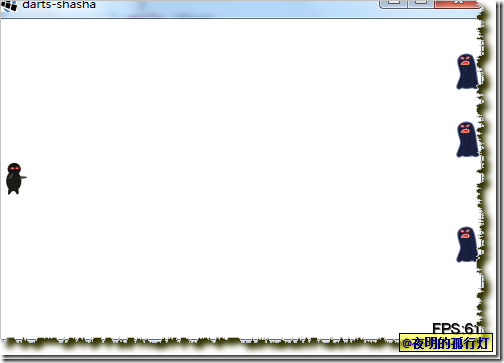```public void AddMove(Actor actor, float time) {
}```

```image.setY(tempY);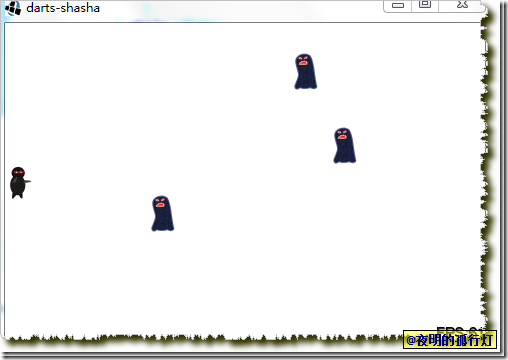# 添加武器

```public static Image createProjectile(AtlasRegion region, Actor man,
Vector3 target) {
Image image = new Image(region);
image.setX(man.getX() + man.getWidth() / 2);
image.setY(man.getY() + man.getHeight() / 2);
return image;
}```

`public class DartsShaSha extends InputAdapter implements ApplicationListener`

```public boolean touchDown(int screenX, int screenY, int pointer, int button) {
Vector3 vector3 = new Vector3(screenX, screenY, 0);
stage.getCamera().unproject(vector3); // 坐标转化
atlas.findRegion("Projectile"), man, vector3)); // 添加新飞镖到飞镖组
return true;
}```

```stage.addActor(projectiles); //添加飞镖组到舞台

InputMultiplexer multiplexer = new InputMultiplexer(); //多输入接收器
Gdx.input.setInputProcessor(multiplexer); //设置多输入接收器为接收器```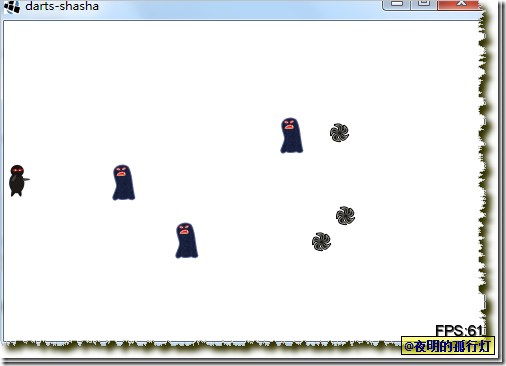# 更完善的飞镖

`image.addAction(Actions.repeat(50, Actions.rotateBy(360, 0.5f))); //设置飞镖的旋转`

```public static Boolean checkAlive(Actor projectile) {
if (projectile.getActions().size == 1) {
return false;
}
return true;
}```

```// 飞镖的移除判
Actor[] projectile = projectiles.getChildren().begin(); //获取Actor数组
for (int j = 0; j < projectiles.getChildren().size; j++) { //移除判断
Actor actor = projectile[j];
if (!ProjectileFactory.checkAlive(actor)) {
projectiles.removeActor(actor);
}
}```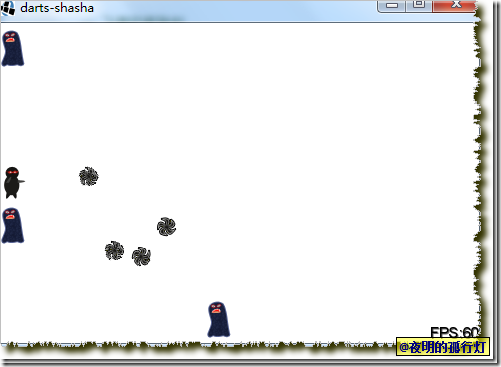`image.setOrigin(image.getWidth() / 2, image.getHeight() / 2);`

# 碰撞检测和杀敌

```public static Boolean attackAlive(Actor target, Actor projectile) {
Rectangle rectangle = new Rectangle(target.getX(), target.getY(),
target.getWidth(), target.getHeight()); // 创建一个矩形
return rectangle.contains(
projectile.getX() + projectile.getWidth() / 2,
projectile.getY() + projectile.getHeight() / 2); //判断是否在矩阵中，即是否击中
}```

```// 开始处理飞镖
Actor[] projectile = projectiles.getChildren().begin();
Actor[] targets = targetGroup.getChildren().begin();
for (int i = 0; i < projectiles.getChildren().size; i++) {
Actor actor = projectile[i];
for (int j = 0; j < targetGroup.getChildren().size; j++) {
Actor target = targets[j];
if (ProjectileFactory.attackAlive(target, actor)) {
targetGroup.removeActor(target);
projectiles.removeActor(actor);
break;
}
}
}

// 如果飞镖已经飞到则刪除
projectile = projectiles.getChildren().begin();
for (int j = 0; j < projectiles.getChildren().size; j++) {
Actor actor = projectile[j];
if (!ProjectileFactory.checkAlive(actor)) {
projectiles.removeActor(actor);
}
}```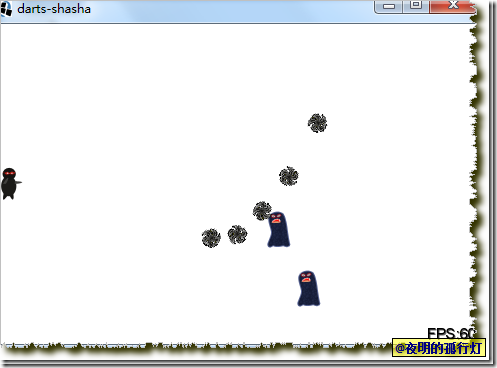# 写在最后

ps：testin的测试结果是通过率 100.00%

ps: 代码上传到github上了，地址 https://github.com/htynkn/DartsShaSha